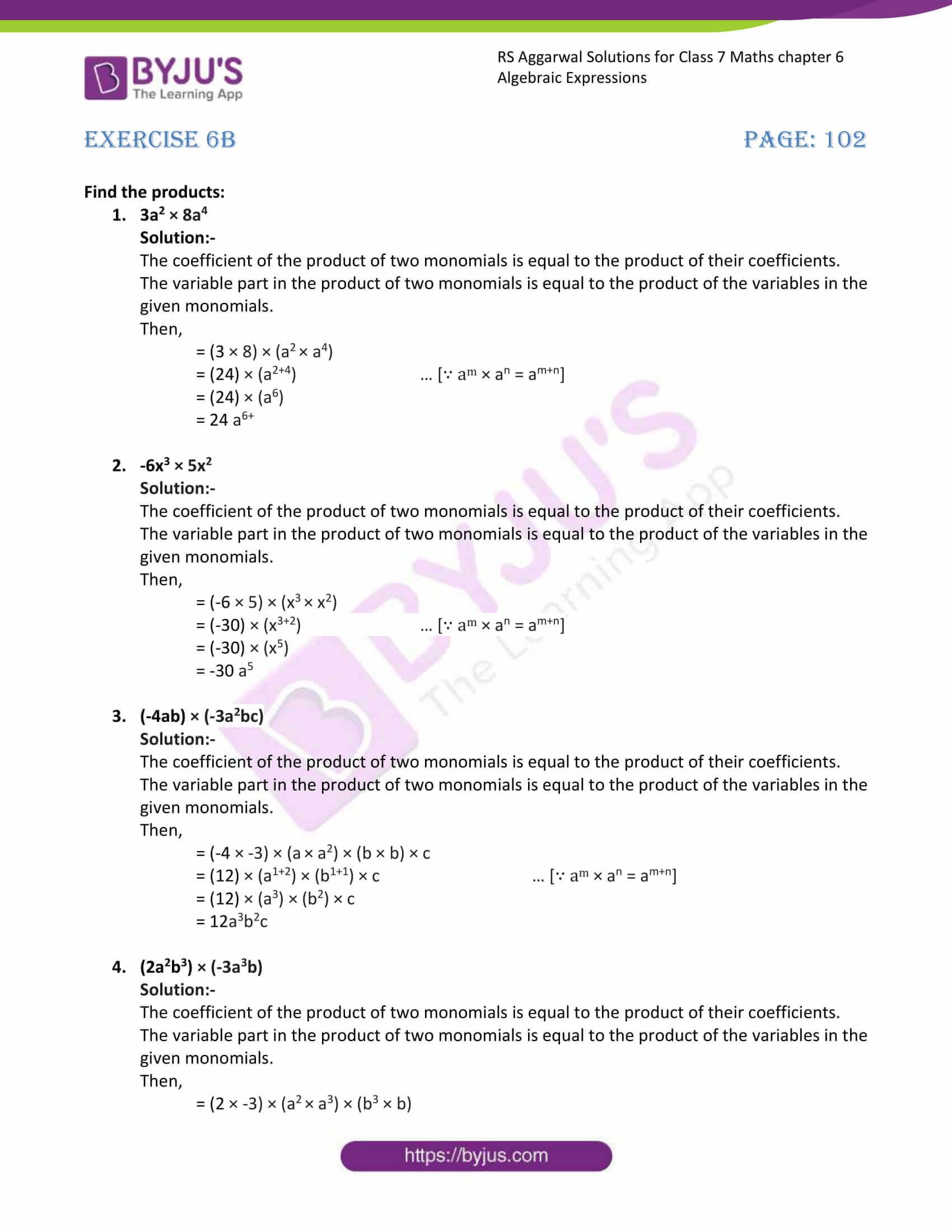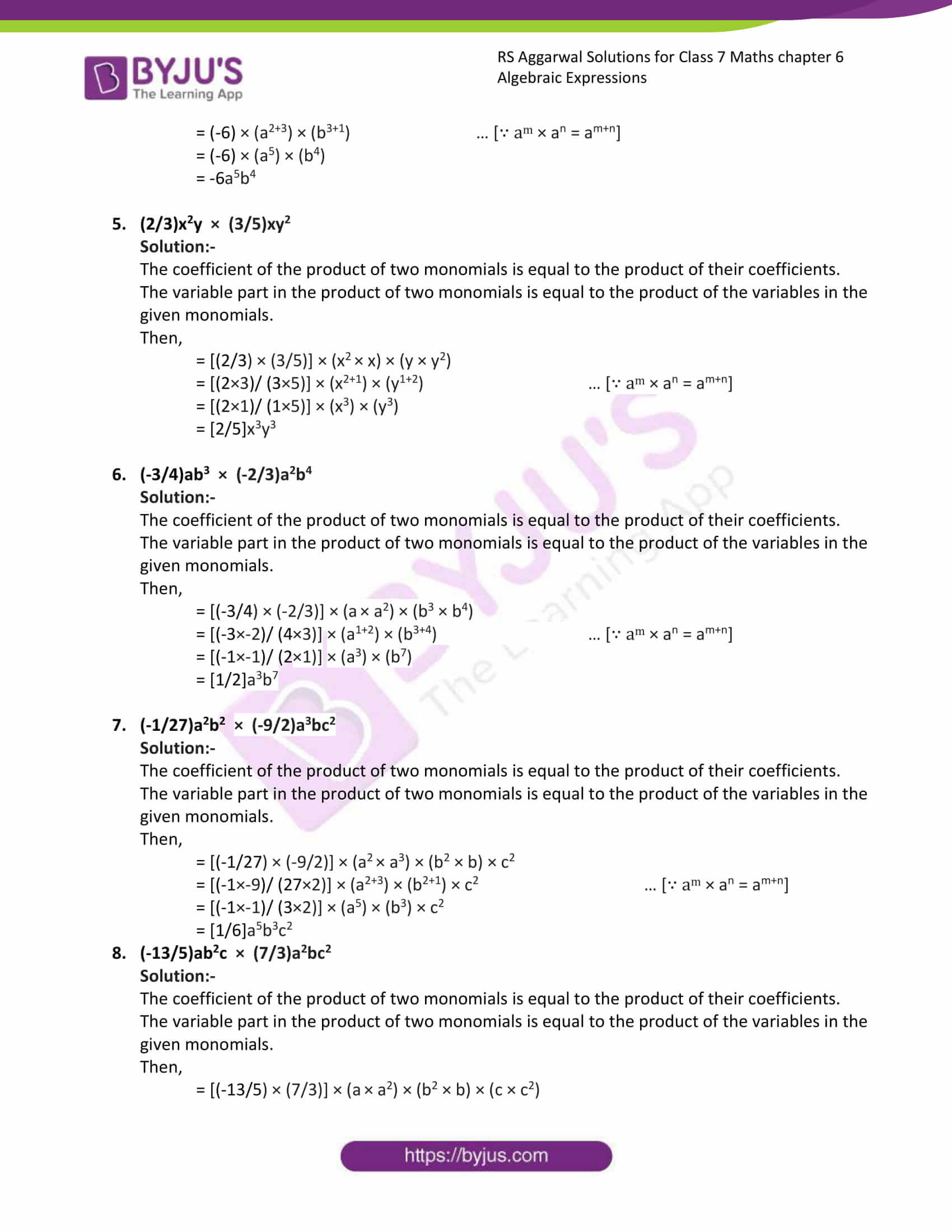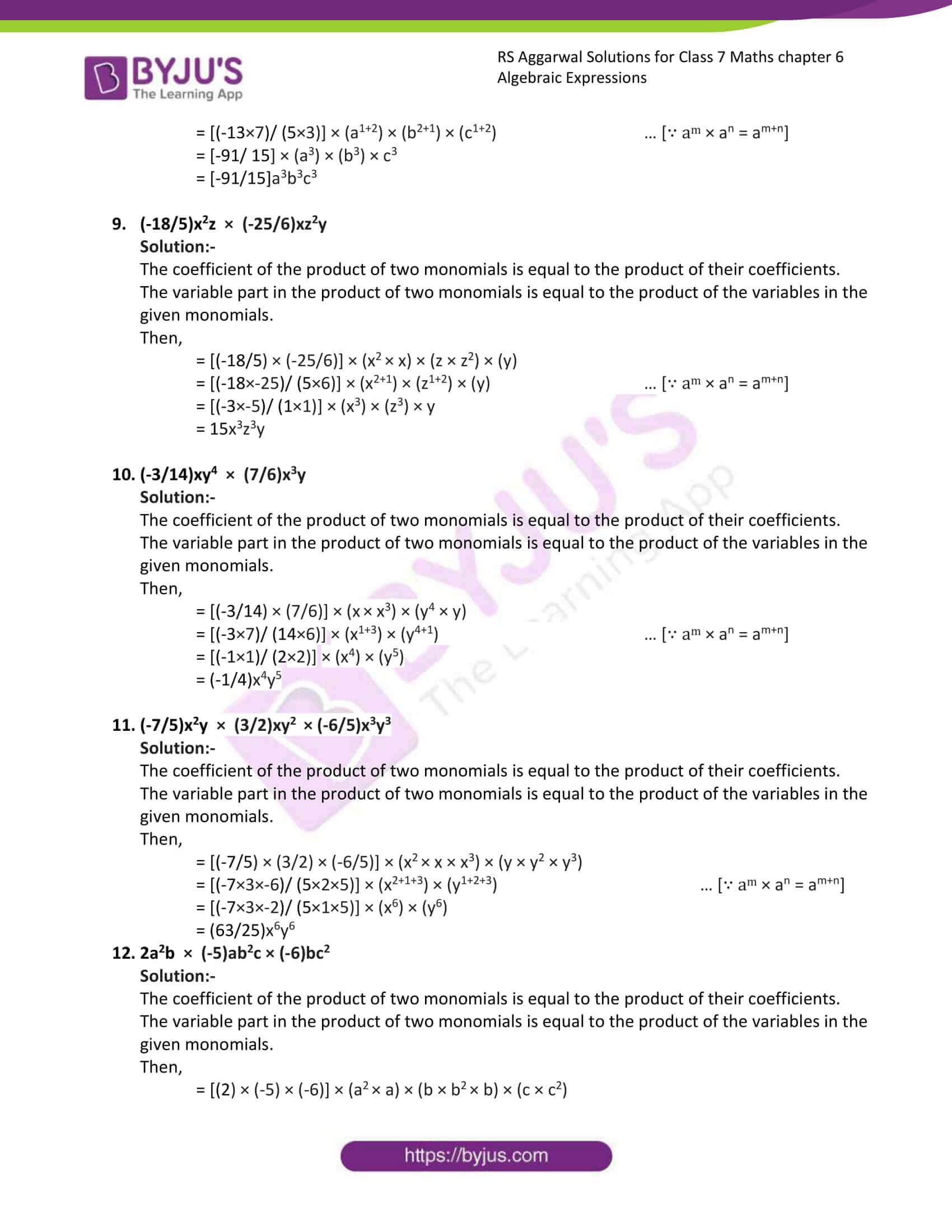# RS Aggarwal Solutions for Class 7 Maths Exercise 6B Chapter 6 Algebraic Expressions

RS Aggarwal Solutions for Class 7 Maths Exercise 6B Chapter 6 Algebraic Expressions in simple PDF are available here. This exercise of RS Aggarwal Solutions for Class 7 Chapter 6 has the questions related to the topic multiplication of algebraic expressions and multiplication of monomials. Our specialist tutors have solved the problems step by step with neat descriptions. By practising RS Aggarwal Solutions for Class 7 Maths Chapter 6 Algebraic Expressions, students can get good marks in Maths.

## Download the PDF of RS Aggarwal Solutions For Class 7 Maths Chapter 6 Algebraic Expressions – Exercise 6B### Access answers to Maths RS Aggarwal Solutions for Class 7 Chapter 6 – Algebraic Expressions Exercise 6B

Find the products:

1. 3a2 × 8a4

Solution:-

The coefficient of the product of two monomials is equal to the product of their coefficients.

The variable part in the product of two monomials is equal to the product of the variables in the given monomials.

Then,

= (3 × 8) × (a2 × a4)

= (24) × (a2+4) … [∵ am × an = am+n]

= (24) × (a6)

= 24 a6+

2. -6x3 × 5x2

Solution:-

The coefficient of the product of two monomials is equal to the product of their coefficients.

The variable part in the product of two monomials is equal to the product of the variables in the given monomials.

Then,

= (-6 × 5) × (x3 × x2)

= (-30) × (x3+2) … [∵ am × an = am+n]

= (-30) × (x5)

= -30 a5

3.(-4ab) × (-3a2bc)

Solution:-

The coefficient of the product of two monomials is equal to the product of their coefficients.

The variable part in the product of two monomials is equal to the product of the variables in the given monomials.

Then,

= (-4 × -3) × (a × a2) × (b × b) × c

= (12) × (a1+2) × (b1+1) × c … [∵ am × an = am+n]

= (12) × (a3) × (b2) × c

= 12a3b2c

4. (2a2b3) × (-3a3b)

Solution:-

The coefficient of the product of two monomials is equal to the product of their coefficients.

The variable part in the product of two monomials is equal to the product of the variables in the given monomials.

Then,

= (2 × -3) × (a2 × a3) × (b3 × b)

= (-6) × (a2+3) × (b3+1) … [∵ am × an = am+n]

= (-6) × (a5) × (b4)

= -6a5b4

5. (2/3)x2y × (3/5)xy2

Solution:-

The coefficient of the product of two monomials is equal to the product of their coefficients.

The variable part in the product of two monomials is equal to the product of the variables in the given monomials.

Then,

= [(2/3) × (3/5)] × (x2 × x) × (y × y2)

= [(2×3)/ (3×5)] × (x2+1) × (y1+2) … [∵ am × an = am+n]

= [(2×1)/ (1×5)] × (x3) × (y3)

= [2/5]x3y3

6. (-3/4)ab3 × (-2/3)a2b4

Solution:-

The coefficient of the product of two monomials is equal to the product of their coefficients.

The variable part in the product of two monomials is equal to the product of the variables in the given monomials.

Then,

= [(-3/4) × (-2/3)] × (a × a2) × (b3 × b4)

= [(-3×-2)/ (4×3)] × (a1+2) × (b3+4) … [∵ am × an = am+n]

= [(-1×-1)/ (2×1)] × (a3) × (b7)

= [1/2]a3b7

7. (-1/27)a2b2 × (-9/2)a3bc2

Solution:-

The coefficient of the product of two monomials is equal to the product of their coefficients.

The variable part in the product of two monomials is equal to the product of the variables in the given monomials.

Then,

= [(-1/27) × (-9/2)] × (a2 × a3) × (b2 × b) × c2

= [(-1×-9)/ (27×2)] × (a2+3) × (b2+1) × c2 … [∵ am × an = am+n]

= [(-1×-1)/ (3×2)] × (a5) × (b3) × c2

= [1/6]a5b3c2

8. (-13/5)ab2c × (7/3)a2bc2

Solution:-

The coefficient of the product of two monomials is equal to the product of their coefficients.

The variable part in the product of two monomials is equal to the product of the variables in the given monomials.

Then,

= [(-13/5) × (7/3)] × (a × a2) × (b2 × b) × (c × c2)

= [(-13×7)/ (5×3)] × (a1+2) × (b2+1) × (c1+2) … [∵ am × an = am+n]

= [-91/ 15] × (a3) × (b3) × c3

= [-91/15]a3b3c3

9. (-18/5)x2z × (-25/6)xz2y

Solution:-

The coefficient of the product of two monomials is equal to the product of their coefficients.

The variable part in the product of two monomials is equal to the product of the variables in the given monomials.

Then,

= [(-18/5) × (-25/6)] × (x2 × x) × (z × z2) × (y)

= [(-18×-25)/ (5×6)] × (x2+1) × (z1+2) × (y) … [∵ am × an = am+n]

= [(-3×-5)/ (1×1)] × (x3) × (z3) × y

= 15x3z3y

10. (-3/14)xy4 × (7/6)x3y

Solution:-

The coefficient of the product of two monomials is equal to the product of their coefficients.

The variable part in the product of two monomials is equal to the product of the variables in the given monomials.

Then,

= [(-3/14) × (7/6)] × (x × x3) × (y4 × y)

= [(-3×7)/ (14×6)] × (x1+3) × (y4+1) … [∵ am × an = am+n]

= [(-1×1)/ (2×2)] × (x4) × (y5)

= (-1/4)x4y5

11. (-7/5)x2y × (3/2)xy2 × (-6/5)x3y3

Solution:-

The coefficient of the product of two monomials is equal to the product of their coefficients.

The variable part in the product of two monomials is equal to the product of the variables in the given monomials.

Then,

= [(-7/5) × (3/2) × (-6/5)] × (x2 × x × x3) × (y × y2 × y3)

= [(-7×3×-6)/ (5×2×5)] × (x2+1+3) × (y1+2+3) … [∵ am × an = am+n]

= [(-7×3×-2)/ (5×1×5)] × (x6) × (y6)

= (63/25)x6y6

12. 2a2b × (-5)ab2c × (-6)bc2

Solution:-

The coefficient of the product of two monomials is equal to the product of their coefficients.

The variable part in the product of two monomials is equal to the product of the variables in the given monomials.

Then,

= [(2) × (-5) × (-6)] × (a2 × a) × (b × b2 × b) × (c × c2)

=  × (a2+1) × (b1+2+1) × (c1+2) … [∵ am × an = am+n]

=  × (a3) × (b4) × (c3)

= a3b4c3

### Access other exercises of RS Aggarwal Solutions For Class 7 Chapter 6 – Algebraic Expressions

Exercise 6D Solutions